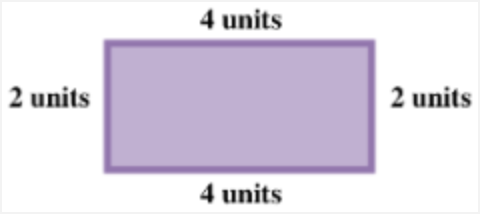### Home > MC1 > Chapter 3 > Lesson 3.2.3 > Problem3-51

3-51.

Draw and label two different rectangles with perimeters of $20$ units each. Do the rectangles have the same area? Explain your thinking. .

Your rectangles should have four sides, and the two sides opposite each other should be the same length.

Remember that the perimeter is the sum of all of the sides of a rectangle.

The area of a rectangle is the number of square units inside of the figure. Try finding the area by multiplying the length of the rectangle by its width.

Now try finding the areas of your rectangles. Are they the same?Perimeter $=4+4+2+2=12$ inches

Your rectangles should not have the same areas because the dimensions are different. This means the products ((length)(width)) will be different as well.

Use the eTool below to draw different rectangles.
Click the link at right for the full version of the eTool: 3-51 HW eTool